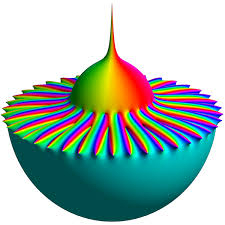# Suppose 5

Suppose z5=2+3i and z6=6+9i are complex numbers and 3 z5 + 7 z6= m+in. What is the value of m and n.

m =  48
n =  72

### Step-by-step explanation:Did you find an error or inaccuracy? Feel free to write us. Thank you!

Showing 1 comment:
Dr Math

Tips for related online calculators